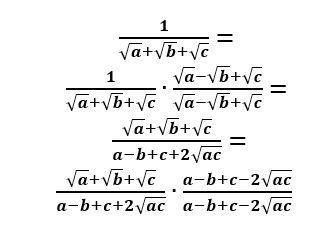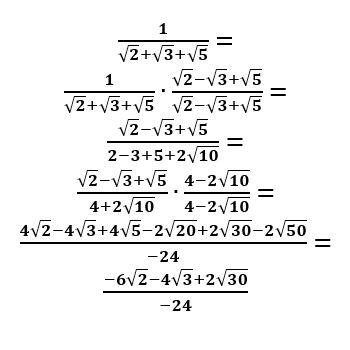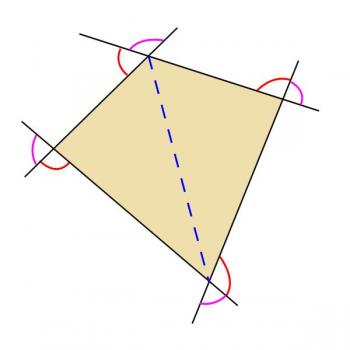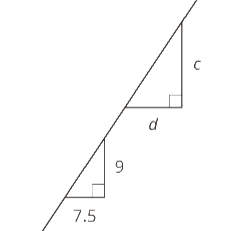Games
Problems
Go Pro!

Category results for 'math'.

Good morning, Larry. Before answering your question, I'd like to give a bit of background on Star Operations, in case someone is not familiar with them. If you're not interested in background, you can skip to the horizontal bar, where I'll try to answer your question.

Star Operations are arbitrarily constructed operations in which a definition is given for how the operation is performed between two variables. They are referred to as "star operations" because the asterisk is often used as the symbol for the operation. For example, here is a star operation definition:

a * b = a2 + b2.

I had a student ask me once what these operations are good for, and my answer was, "They're good for teachers who like to torment students." That was, of course, a bit tongue-in-cheek, but the primary purposes they serve are:

• Giving students practice with arithmetic/algebraic manipulation
• Introducing the concept of functions, without actually using the word or symbolism of functions. The star operation given above can also be written as:

f(a, b) = a2 + b2

With that as background, let's get to Larry's question.

For the sake of this discussion, let's use the star operation given above: a * b = a2 + b2. Larry wants to know if the distributive property works for star operations, so let's give it a try and see what happens. We kind of need to guess how a distributive property would be implemented - there are several possibilities, which I'll list below:

1. a(x * y) = ax * ay
2. a * (x + y) = a * x + a * y
3. a * (x * y) = (a * x) * (a * y)

In the first case, the addition was replaced with a star. In the second, the multiplication was replaced with a star, and in the third, both the addition and the multiplication were replaced with a star. Let's test each of these by plugging in values for a, x, and y. If the values we pick work, that doesn't prove that the rule is legitimate, but if the values don't work, we know that the rule is bogus. Let's do the following values:

a = 3; x = 4; y = 2.

## Possibility #1

3(4 * 2) = 3(4) * 3(2)
3(16 + 4) = 12 * 6
3(20) = 144 + 36
60 = 180, which is NOT true, so the first possibility is bogus

## Possibility #2

3 * (4 + 2) = 3 * 4 + 3 * 2
3 * 6 = (9 + 16) + (9 + 4)
9 + 36 = 25 + 13
45 = 38;  also bogus!

## Possibility #3

3 * (4 * 2) = (3 * 4) * (3 * 2)
3 * (16 + 4) = (9 + 16) * (9 + 4)
3 * 20 = 25 * 13
9 + 400 = 625 + 169
409 = 794; BOGUS!

So we tried all the possibilities, and none of them worked. That doesn't mean that they are bogus for all star operations. However, it doesn't work for all star operations, it's not really good for much, is it?This morning's question is, in essence, if you know the probability of an event happening, what is the probability of it NOT happening?

To answer this question, it's good to remember that a probability is a ratio. It is: [number of desired outcomes] : [total number of outcomes] (assuming that all outcomes are equally likely).

Let's consider the flipping of a coin. Suppose you want the probability of getting heads. The number of desired outcomes is 1. The total number of outcomes is 2.*

'So the probability is 1/2. So what is the probability of NOT getting heads? Well, that's the same as the probability of getting tails. Desired outcomes: 1, possible outcomes 2. Thus, the probability is also 1/2.

* I've had students argue that a coin could land on its rim, so there are actually 3 possible outcomes. I point out to them that there are a couple problems with that. First, if it lands on the rim, we re-roll the coin, so that really doesn't count. Second, if we decided to keep that roll, we can't use our ratio definition above, because all outcomes are not equally likely.

So now let's switch gears and talk about a six-sided die. What is the probability of getting a perfect square when you roll the die? Well, there are 2 perfect squares that are possible results: 1, and 4. So desired outcomes = 2, total outcomes  = 6. Thus the probability is 2/6 (we can simplify that to 1/3, but for now I'd like to keep in the form 2/6).

What is the probability of NOT getting a perfect square? Well, there are 4 numbers that aren't perfect squares: 2, 3, 5, and 6. Desired outcomes = 4, total outcomes = 6. Probability is 4/6.

Let's try one more example. What is the probability of rolling a total of 12 if you roll two six-sided dice?

There is only one way to get a sum of 12: you need a six on each die. There is a total of 6 x 6 = 36 possible outcomes. Desired = 1, Total = 36, probability = 1/36. What about the probability of NOT rolling a 12? Well, you could list off all the possible outcomes, but let's reason this out instead. If there are 36 possible outcomes, and only one of them leads to a sum of 12, how many don't lead to a sum of 12? The answer should be fairly obvious: 36 - 1 = 35. Desired = 35, total = 36, probablity = 35/36.

Now let's put those results together:

Coin: 1/2; 1/2
Die: 2/6; 4/6
2 Dice: 1/36; 35/36

Hopefully you can see a pattern here: in each case, the probabilities add to 1. And, if you think about how we reasoned out the example with two dice, that should make sense to you; every outcome is either a desired or a not desired outcome. There are no other options. So when you add together those options, you get the total number of outcomes. And [total outcomes]/[total outcomes] = 1.

We can write this rule as an equation like this: P(x) + P(~x) = 1.

In words: The probability of x, plus the probability of NOT x equals 1.

I hope that helps!

Question from Federico: "How do you rationalize the denominator of a fraction if there are three radicals added together, like SQR(2) + SQR(3) + SQR(5)?"

That's an interesting question, and to be completely honest, it's not one I remember coming across before. So I had to play around with it to see what I could find out. I lucked out, though, and the first thing I tried seemed to work out pretty well.

I started out by using variables instead of numbers, to see if I could find something that would always work.  I'll show you the work I did below.So what did I do? I replaced one of the plus signs with a minus, and multiplied numerator and denomator by that, similar to how we would multiply by the conjugate if there were just two terms. This caused all the radicals to disappear except the square root of ac, and that brings us to our normal simplification process.

So here's how it looks with your particular example:You can check on a calculator to see that the original value and my final "rationalized" value are the same thing.

Really, though, it doesn't look any simpler, and it was probably more effort than it was worth - but at least I know how to do it now - thanks for asking!

NOTE: I was so focused on rationalizing the denominator that I didn't even think about simplifying further. Of course, you know how to do that, and can finish the process for me!

Chanchal from Muktsar asks if we could prove that in a quadrilateral the sum of exterior angles is 360°.

Good morning, Chanchal. One of the challenges of doing proofs on this blog is, a proof is constructed from the building blocks of things we already know, stacked together to create something we don't already know, and since I don't know you, I don't know what building blocks (knowledge) you have that you can build from. So before I start talking through the proof, here are some of the building blocks I'm going to use - in case you don't already know these things:

• the sum of the interior angles in a triangle is 180°
• when two lines intersect, they form four angles that add to 360°.
• vertical angles are congruent (vertical angles are the angles across from each other formed by two intersecting lines)

Okay, with that as background, let's look at a diagram.Now, my diagram is not just a quadrilateral - I've added some extra lines into it.

• The blue dashed line is a diagonal of the quadrilateral
• The sides of the quadrilateral have been extended to form exterior angles
• The purple arcs indicate angles which are opposite (vertical) to the interior angles of the quadrilateral
• The red arcs indicate the angles we're interested in.

Note that when we talk about the exterior angles of a quadrilateral, we're not talking about all the angles formed by the sides that lie outside the quadrilateral. We're not including the purple angles, and we're also not including the angles opposite the red ones. The exterior angles are all the angles "facing the same way" around the quadrilateral. That's not a very precise way of describing them, but hopefully you can see from my picture what I mean by that.

Okay, so how do we prove this? I'll give you two methods, and you can decide which one you like best. Ready?

## Proof #1

• A quadrilateral can be divided into two triangles by a diagonal
• Each triangle has 180 degrees
• 180 x 2 = 360, so there are 360 degrees in the interior of a quadrilateral.
• Call these four angles a, b, c, and d. Then a + b + c + d = 360
• Each angle is supplementary to an exterior angle.
• Thus, the exterior angle measures are 180 - a, 180 - b, 180 - c, and 180 - d
• Adding these together gives (180 - a) + (180 - b) + (180 - c) + (180 - d) = 720 - (a + b + c + d)
• Since a + b + c + d = 360, this is equal to 720 - 360, which equals 360 degrees.

## Proof #2

(Proof #2 starts out with some of the same steps as Proof #1)

• A quadrilateral can be divided into two triangles by a diagonal
• Each triangle has 180 degrees
• 180 x 2 = 360, so there are 360 degrees in the interior of a quadrilateral.
• Call these four angles a, b, c, and d. Then a + b + c + d = 360
• The intersecting lines at the four vertices form angles adding to 360 degrees
• Since there are four such sets of angles, their measures add to 360 x 4 = 1440 degrees.
• The purple angles from vertical pairs with the interior angles, so their measures are a, b, c, and d
• Thus, the sum of the red angles and their vertical counterparts is 1440 - (a + b + c + d) - (a + b + c + d) = 720 degrees
• Since vertical angles are congruent, we divide this sum in half to obtain the sum of the red angles: 720 / 2 = 360 degrees.

Incidentally, this proof can be extended to show that this is true not just for quadrilaterals, but for any polygon; the sum of the exterior angles is 360 degrees, regardless of the number of sides.  Thanks for asking, Chanchal!

"If the two triangles shown are similar, find the value of d/c. I know the answer is 5/6, but I don't know how to get it." ~KimberlyGood morning Kimberly. As we look at this question, I'd like to point out that it does violate one of our standard rules of geometry - which is that we never assume the diagrams are drawn to scale. The problem writer clearly intends for you to assume that the lines which appear to be horizontal really are horizontal, and the ones that appear to be vertical really are vertical. If you make that assumption, then you can conclude that the side of length 7.5 corresponds to the side of length d, and the side of length 9 corresponds to the side of length c. So we'll go with that assumption.

Since the triangles are similar, and we "know" which sides are corresponding, we can set up a ratio:

7.5/d = 9/c.

This does not give us what we want (d/c). However, we can rearrange that with just a couple steps to obtain the desired result. First multiply both sides by d, to get it in the numerator:

d(7.5/d) = d(9/c)
7.5 = 9d/c

Now divide both sides by 9:

7.5/9 = d/c

This is the ratio you want, except that it's not in simplest form. Multiply numerator and denominator by 2 in order to get rid of the decimal:

7.5/9 = 15/18 = 5/6.

And there's your answer. But I'm not quite done - I'd like to point out something that might be valuable to you. This problem shows us something very interesting that I often make use of when doing similar triangle problems.

We started with a ratio of corresponding sides, and when we were done, we ended with a very different ratio - the ratio of sides within a triangle.

Your standard rule is: The ratios of corresponding sides in two similar triangles are equal.

Our alternate version (which you can call the Kimberly Rule, if you want) is: The ratio of any two sides in a triangle is equal to the ratio of the corresponding sides in a second, similar triangle.

In other words, you could have started out with d/c = 7.5/9 instead of starting out with 7.5/d = 9/c.

Older posts

# Featured Resources on This Site

Create Christmas ornaments and more
Print fun mazes in many different shapes

# Blogs on This SiteReviews and book lists - books we love!The site administrator fields questions from visitors.Like us on Facebook to get updates about new resources